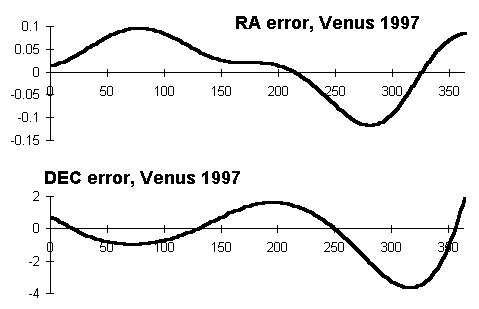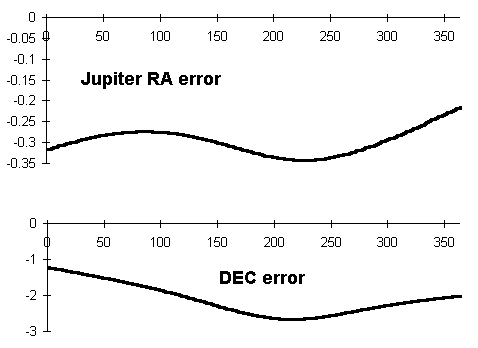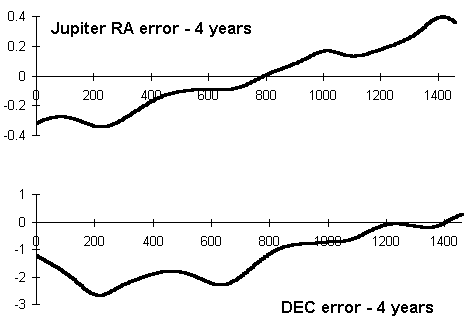# Planet positions using circular orbits

[Root ]

## Overview

[Top]

"With them it is as though an artist would gather the hands, feet, head and other members for his images from diverse models, each part excellently drawn, but not related to a single body, and since they in no way match each other, the result would be a monster rather than a man."

Copernicus

This page is concerned with astronomical calculations. You can find out how to calculate a very rough position (given as the right ascension and declination) for the planet Venus, and for the planet Jupiter on any given day. The method will extend to any of the other planets, and is accurate to a few degrees either way in the sky - enough for binoculars! As an example of the method, I show calculations for the position of Jupiter and of Venus at 0 UT on April 20th/21st, 1997.

The method shown here is based on Section 55 of Peter Duffett- Smith's book Practical Astronomy with your Calculator (3rd Edition, 1990). You can plot your calculated position on a star chart - or convert to ALT and AZ coordinates and find the planets with a magnetic compass.

If you want accurate positions for the planets until 2006, I would suggest you look at the NASA Twelve Year Planetary Ephemeris (TYPE), provided by Fred Espenak. I have used this very useful resource to check the accuracy of the method described here.

## Method

[Top]

The planets orbit the Sun following paths which are approximately elliptical. The planes of their orbits are tilted to the plane of the Ecliptic by varying small angles. Both these things complicate the calculation of the position of the planet. In the following calculations, we make two outrageous assumptions;

• Orbits are circular
• All orbits lie in the plane of the Ecliptic
Having made these radical assumptions (which would probably cause Kepler to gyrate in his grave), we can then break down the calculations into three broad stages;
• Find the position of the Earth in its orbit (step 1 below),
• Find the position of the planet in its orbit (step 2 below),
• Refer the position of the planet to the Earth (steps 3 to 5 below),
• Convert the position to RA and DEC (step 6 below).

To find the position of the planet in its orbit today, we just need to know its position at some instant in time (known as an epoch) and how fast the planet is moving. This kind of information is listed for various dates in the Astronomical Ephemeris, and in Duffett-Smith's book. A set of 7 numbers define a proper eliptical orbit - and these are called the elements of the orbit. I list just the elements needed for this simplified calculation in the 'Elements' section below.

As we have assumed that the planes of the orbits are the same as the plane of the Ecliptic, a simple sum gives us the Heliocentric Longitude of the planet. The Heliocentric Latitude of the planet is assumed to be zero throughout. We also need to find the Heliocentric Longitude of the Earth, to allow the positions to be referred back to the Earth in the next step.

Having found the position of the planet and of the Earth as angles measured from the Sun, we need to refer that position to the Earth. This requires some formulas from trigonometry. We end up with the Geocentric Ecliptic Longitude of the planet - again the Geocentric Ecliptic Latitude of the planet is zero as a result of our assumptions.

Star maps and Planispheres are usually calibrated in Equatorial Coordinates, consisting of the right ascension and declination. We need to convert our Geocentric Ecliptic Longitude to RA and DEC. You can then either;

• plot the positions on a star map
• convert the RA and DEC to ALT and AZ and use a compass to find the planets (add 6 degrees on to the AZ for the magnetic deflection).

As the results of these calculations are of low accuracy, there is little point in correcting for light travel time or for planetrary aberration. See Duffett-Smith's book for more accurate methods and for corrections such as these.

## Elements of Orbits

[Top]

We only need the Mean longitude at epoch (L) and the daily motion (n) for the planet and for Earth, and the semi major axis of the orbit (a) of the planet - which we regard as being the radius of the circular orbit. The values below are from the 1996 Astronomical Ephemeris;

```Epoch JD 2450320.5 - 0h UT 25th August 1996

Planet         n           L            a
Mercury    4.092385     281.18017   0.3870975
Venus      1.602159      20.17002   0.7233235
Earth      0.9855931    333.58600   1.0000108 (taken as 1)
Mars       0.5240218     73.77336   1.5237131
Jupiter    0.08310024   292.64251   5.202427
Saturn     0.03333857     8.91324   9.561943
Uranus     0.01162075   298.87491  19.30425
Neptune    0.005916098  297.58472  30.27750

n - daily motion in degrees per day
L - Mean Longitude or Longitude at Epoch (degrees)
a - Mean distance (au) - taken as radius of orbit here
```

I tried the calculations using the 1990.0 epoch elements given by Duffett-Smith in the current printing of Practical Astronomy with your Calculator, and the differences were very small compared to the error overall. The book can thus claim to be self contained, you won't need to go to a library to get new figures.

## Position of Earth in its orbit

[Top]

As we assume that all the orbits are circular, the planets travel round them at an even angular speed - we often see the 'mean daily motion' listed in degrees per day. To find the Heliocentric Longitude of the Earth a given number of days later than the epoch of the elements, we just multiply the number of days by the daily motion, and add on the Longitude it had at the epoch of the elements. Just as an (analogue) clock goes to zero when it reaches 12 - we have to subtract off multiples of 360 degrees to bring the Longitude back into the range 0 to 360.

The heliocentric longitude of the Earth is given by;

```hle - is the heliocentric longitude of the Earth
ne  - is the daily motion of the Earth
D   - is the number of days since the epoch of the elements
Le  - is the longitude of the Earth at the epoch of the elements

1) hle = ne * D + Le

Subtract multiples of 360 to bring into range 0-360 degrees
```
On April 20th 1997,
``` ne = 0.9855931
D = 128 + 31 + 28 + 31 + 20 = 238 days since epoch of elements
Le = 333.58600

1) hle = 0.9855931 * 238 + 333.58600
= 568.1571578 - 360
= 208.1571578 degrees
```
This value is used to refer the positions of Venus and Jupiter back to the Earth.

## Venus (inner planet)

[Top]

To find the geocentric longitude of Venus, we find the heliocentric longitude of the planet (step 2), and then change our origin of coordinates to the Earth (steps 3 to 5). The formula for referring to the Earth's position is different for inner and outer planets.

### Position of Venus in its orbit

By the same argument as for the Earth, the position of Venus in its orbit can be found from the following formula;
```2) hlv = nv * D + Lv

Subtract multiples of 360 to bring into range 0-360 degrees

hlv - is the heliocentric longitude of Venus
nv  - is the daily motion of Venus
D   - is the number of days since the epoch of the elements
Lv  - is the longitude of Venus at the epoch of the elements

On April 20th 1997,

nv = 1.602159
D = 128 + 31 + 28 + 31 + 20 = 238 days since epoch of elements
lv = 292.64251

2) hlv = 1.602159 * 238 + 20.17002
= 401.483862 - 360
= 41.483862 degrees
```

### Referring to Earth's position

The formulas below transforms the longitude of Venus from the Sun as centre to the Earth as centre. The formulas only work for planets inside the Earth's orbit.
```Recall that,

av - radius of Venus orbit
hle and hlv - the heliocentric longitudes of Earth and Venus
glv - the geocentric longitude of venus

3) calculate;

Y = av * sin(hle - hlv)     (always in range -0.75 to 0.75)
X = 1 - av * cos(hle - hlv) (always in range 0.2 to 1.8)

4) Then find atan(Y/X). If Y is negative, then add 360 to the result
of atan(Y/X), X is always positive.

5) Then

glv = 180 + hle + atan(Y/X)

subtract or add multiples of 360 as needed to bring into
range 0-360

The geocentric latitude of venus is assumed to be zero.
```
Below are the figures for April 20th 1997,
``` av =   0.7233235
hle = 208.1571578
hlv =  41.483862
glv = the geocentric longitude of Venus

3) Y = 0.7233235 * sin(208.1571578 - 41.483862)
= 0.1667284448

X = 1 - 0.7233235 * cos(208.1571578 - 41.483862)
= 1.703845517

4) atan(Y/X) = 5.5588839611 degrees (no correction, as Y positive)

5) glv = 180 + 208.1571578 + 5.5588839611
= 393.74599741 - 360
=  33.74599741 degrees
```

You can skip to the section on transforming to RA and DEC to get the equatorial coordinates for Venus, or carry on to calculate the geocentric longitude for Jupiter.

## Jupiter (outer planet)

[Top]

To find the Geocentric longitude of Jupiter, we find the heliocentric longitude of the planet (step 2), and then change our origin of coordinates to the Earth (steps 3 to 5). The formula for referring to the Earth's position is different for inner and outer planets.

### Position of Jupiter in its orbit

By the same argument as for the Earth, the position of Jupiter in its orbit can be found from the following formula;
```2) hlj = nj * D + Lj

Subtract multiples of 360 to bring into range 0-360 degrees

hlj - is the heliocentric longitude of Venus
nj  - is the daily motion of Venus
D   - is the number of days since the epoch of the elements
Lj  - is the longitude of Venus at the epoch of the elements
```
On April 20th 1997,
``` nj = 0.08310024
D = 128 + 31 + 28 + 31 + 20 = 238 days since epoch of elements
lj = 292.64251

2) hlj = 0.08310024 * 238 + 292.64251
= 312.4203671 degrees
```

### Referring to Earth's position

The formula below transforms the longitude of jupiter from the Sun as centre to the Earth as centre. The formula only works for planets outside the Earth's orbit.
```Recall that,

aj - radius of Jupiter's orbit
hle and hlj - the heliocentric longitudes of Earth and Jupiter
glj - the geocentric longitude of Jupiter

3) calculate;

Y = sin(hlj - hle)         (always in range -1 to 1)
X = aj - cos(hlj - hle)    (always in range 4.2 to 6.2)

4) Then find atan(Y/X).
If Y < 0 then add 360 to result - X is always positive

5) Then

glj = hlj + atan(Y/X)

subtract or add multiples of 360 as needed to bring into
range 0-360

The geocentric latitude of Jupiter is assumed to be zero.
```

For April 20th 1997, we have

``` aj =   5.20247
hle = 208.1571578
hlj = 312.4203671
glj = the geocentric longitude of Jupiter

3) Y = sin(312.4203671 - 208.1571578)
= 0.9691741343

X = 5.20247 - cos(312.4203671 - 208.1571578)
= 5.448846739

4) atan(Y/X) = 10.08559526 degrees (no correction, as Y positive)

5) glj = 312.4203671 + 10.08559526
= 322.5059624 degrees
```
In the (outrageous) approximation we are using, the Geocentric Latitude of the planet is zero - because we assume that Jupiter's orbit is in the plane of the Ecliptic.

## Transform to RA and DEC

[Top]

In the previous sections we found the Geocentric Longitude (glp) and Latitude of the planets Venus and Jupiter. In the (radical) approximation we are using, the Geocentric Latitude for both planets is zero - as the orbits are assumed to be in the plane of the Ecliptic. We now want to find the Equatorial coordinates of the planets - the right ascension (RA) and declination (DEC). We use the formulas below, and use the value 23.43928 degrees for the inclination of the Equator to the Ecliptic (E).

```E   - is the angle of the Equator to the Ecliptic, and
glp - is the Geocentric Longitude of the planet,
glv for Venus and glj for Jupiter

6) Y = sin(glp)*sin(E)

X = cos(glp)

ra' = arctan(Y/X)

If X < 0 then ra = ra' + 180
If Y < 0 and X >> 0 then ra = ra' + 360
else ra = ra'

DEC = ARCSIN(sin(glp)*sin(E))
```

### Results for Venus

For 20th April 1997, we have the following values from steps 2 to 5 for Venus;
```
E =  23.43928
glv =  33.74599741
```

Substituting these values into the formulas above gives;

```6) Y = sin(33.74599741) * cos(23.43928)
= 0.5096724726

X = 0.8315084215

ra' = arctan(Y/X) = arctan(0.6129492612)
= 31.50618405 degs
no correction as X and Y both positive

ra  = 31.50618405/15
= 2.10041227 hrs

DEC = ARCSIN(sin(33.74599741) * sin(23.43928))
= 12.76603228
= 12.77 degrees
```
TYPE gives RA = 2.16 hrs and DEC = 12.21 degrees for Venus on this day.

### Results for Jupiter

For 20th April 1997, we have the following values from steps 2 to 5 for Jupiter;
```  E =  23.43928
glj = 322.5059624
```

Substituting these values into the formulas above gives;

```6) Y = sin(322.5059624) * cos(23.43928)
= - 0.5584519886

X = cos(322.5059624)
= 0.7934166858

ra' = arctan(Y/X) = arctan(-0.7038571265)
= -35.14007177 degs

add 360 to ra', as Y is negative and X positive

ra  = -35.14007177 + 360 = 324.8599282
= 21.65732855 hrs

DEC = ARCSIN(sin(322.5059624) * sin(23.43928))
= -14.01160621 degrees
= -14.01
```

TYPE gives RA = 21.38 hrs and DEC = - 15.94 degrees for Jupiter on this day.

## Accuracy of method

[Top]

The very crude method outlined here will give you positions for Venus within 0.1 hours of RA and around 3 degrees of DEC. For Jupiter, the RA is only good for 0.4 hours (nearly 6 degrees on the sky) and about 3 degrees in DEC. This level of accuracy is sufficient to put the planet in the right constellation - and within the correct binocular field (7.3 degrees) in most cases.

I wrote a simple BASIC program to repeat the estimated method for every second day in 1997 - and I compared my results with data transferred from the NASA Twelve Year Planetary Ephemeris, using a spreadsheet. The error in each measurement is defined as

```error = TYPE value - estimated value
```

I was expecting to see a double periodicity in the error, one for the Earth's departure from circular motion, and another corresponding to the planet's eccentricity. The graphs for Venus in 1997 confirmed this general picture.The main features of the error in the RA and DEC for Venus in 1997 are;

• The maximum error in RA is -0.15 hours
• There is a large amplitude periodic term with a period of 200 days in the RA error curve
• There is a smaller term super-imposed on the larger one.
• The DEC error reaches a maximum of -4 degrees at 315 days into 1997
• The error curve for DEC also seems to consist of a large amplitude periodic term of period 200 days, but this time the second term is of larger amplitude, which alters the shape of the curve.
The results for Jupiter will be different as a result of the planet's much longer orbital period.The main features of the error in the RA and DEC for Jupiter in 1997 are;

• The maximum error in RA is -0.35 hours
• The RA curve has a large negative error - probably part of a longer term cycle related to Jupiter's 12 orbital period
• There is a smaller term super-imposed on the larger one, possibly part of the Earth's cycle.
• The DEC error reaches a maximum of -3 degrees at 210 days into 1997, but the DEC curve is much less 'corrugated' than the DEC curve for Venus, as you would expect from the longer orbital period.
• A longer part of Jupiter's 12 year cycle should be investigated (back to Excel 8-)

Plotting the errors over 4 years (1997 to 2000 inclusive) shows more of the picture;The main features of the error in the RA and DEC for Jupiter over the period 1997 to 2000 inclusive are;

• The maximum error in RA is -0.4 hours at the beginning of the period, increasing to +0.4 hours at the end of the four years.
• We can se that the RA error has a long period cycle.
• There is a smaller term super-imposed on the larger one, possibly part of the Earth's cycle.
• The DEC error reaches a maximum of -3 degrees at 210 days into 1997.
• The DEC error increases through zero near the end of the 4 year period - there is a long period term here.

Some simple periodic adjustment term may work for Jupiter over the whole 12 year TYPE period - but then you might as well try some interpolation scheme using the TYPE values themselves.

## References

[Top]

A copy of Practical Astronomy with your Calculator by Peter Duffett-Smith provides details on more accurate orbital calculations which are feasible on a programmable calculator. You don't always want to boot up a PC to work out a rough azimuth for a comet - or to see how far below the horizon the Sun will be at a certain time. Here is the full reference;

by Peter Duffett-Smith
Cambridge University Press
ISBN 0-521-35699-7 (3rd Edition)